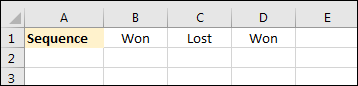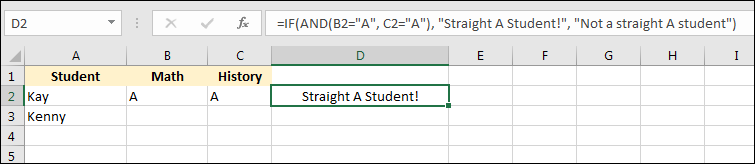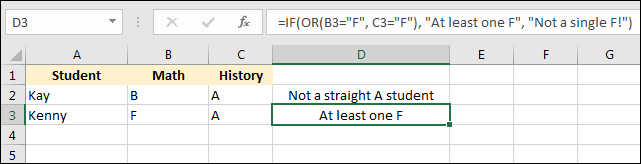# Using AND and OR in Excel IF Statements

The Excel function AND takes a least two values and tells you whether or not they are true. As a simple example, take a sequence like this:You can use AND to see if this sequence is all "Won". Enter the following formula in cell E1:

=AND(B1="Won", C1="Won", D1="Won")

When you press the enter key on your keyboard, cell E1 will have a value of False. It's False because the AND function is checking each cell for a value of "Won". If just one of these values is not "Won" then the whole AND function returns false. If you change the value in cell C1 to Won and press the enter key, cell E1 will have a value of True.

With the AND function, each test for true or false needs to be separated with a comma.

### The Excel OR Function

Similar to AND, but if just one of your tests is true then Excel return true for the whole OR function.

### Using AND/OR in IF Statements

AND and OR are more useful when used with IF Statements. Try this. Create the simple spreadsheet below:We first want to test if a student got straight As. If so, we'll display a suitable message in the D column.

Click inside cell D2. Enter the following formula:

=IF(AND(B2="A", C2="A"), "Straight A Student!", "Not a straight A student")

Now press the enter key on your keyboard. You see that cell D2 contains the text "Straight A Student!". Your spreadsheet should look like this:Now click inside cell B2. Change the grade from A to B. Press the enter key again and cell D2 will change to "Not a straight A student":So how does it work? The first part of the IF Statement uses the AND function:

AND(B2="A", C2="A")

This just checks cells B2 and C2 for the text "A". (If you're checking for text values, you have to surround the text with double quotes.) If both of these are true, if cells B2 and C2 do indeed contains the text "A", then the whole of the AND part evaluates to TRUE. And if the first part of an IF Statement is TRUE then Excel uses whatever you have after the first comma. For us, this was just some text, "Straight A Student". If the AND part is FALSE, then Excel uses whatever you have after the second comma. After our second comma, we have the text "Not a straight A student". Excel is doing this:

If AND(B2="A", C2="A") evaluates to TRUE Then

Display "Straight A Student"

If AND(B2="A", C2="A") evaluates to FALSE Then

Display "Not a straight A Student"

Let's try the OR function. Click in to cell B3. Enter a capital letter F. In cell C3 enter a capital letter A. In cell D3, add the following formula:

=IF(OR(B3="F", C3="F"), "At least one F", "Not a single F!")

Your spreadsheet should look like this:This time, we've used OR as the test for our IF Statement. Now, only one of the conditions needs to be TRUE for the whole OR statement to evaluate as TRUE. If the OR statement is TRUE then the part after the first comma gets executed by Excel. This was to display the test "At least one F". If neither cell B3 or cell C3 contains a letter F then the part after the second comma gets executed. This was the text "Not a single F!".

Try it out. Change the F to a B, for example. You should see the text in cell D3 change.

In the next lesson, we'll explore Conditional Formatting. This is about colouring cells depending on their values. Looks nice on a spreadsheet!

<--Back to the Excel Contents Page